Exercises

In this lesson, kids are given different strategies for finding the sum of two numbers. Addition strategies such as these help third graders gain a deeper understanding of, and fluency with, this operation. The lesson features guided instruction and plenty of practice problems to apply what is being learned. For even more practice, try our third grade addition worksheets recommended to accompany this lesson.
This lesson includes 5 printable learning activities.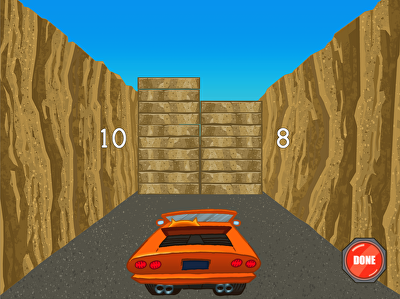Roly is going on a road trip, and wants you to join! In this car-themed game, kids must blast through road blocks with a laser to help Roly keep cruising. In order to bust up obstacles, players must regroup addition problems within 20. Excellent for visual learners, this game helps kids concretely understand how to use the strategy of making ten to simplify addition problems.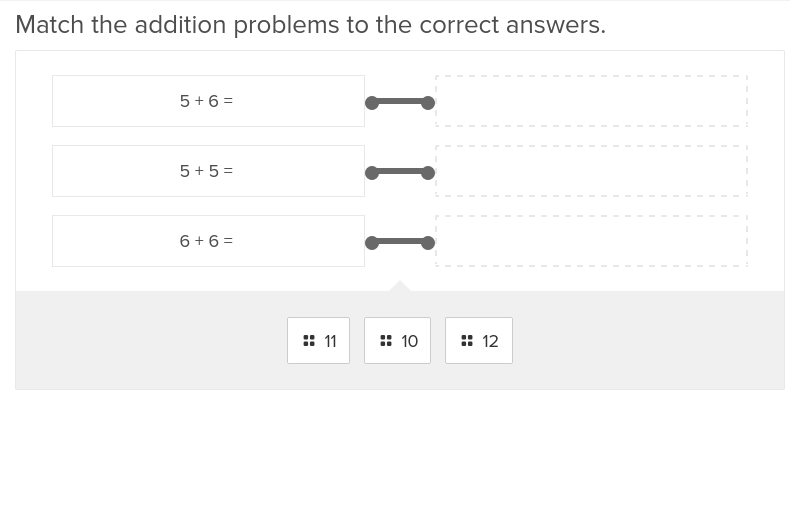Limit addition to within 20 for students new to two digit addition in this engaging exercise.

## Two- and Three- Digit Addition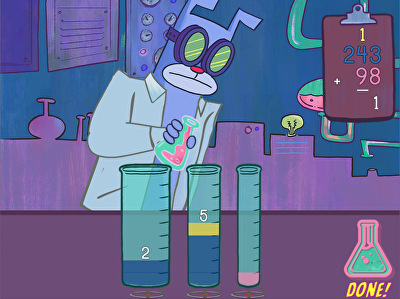Two- and three-digit addition is the focus of this game, featuring Professor Beardo. Students will help him mix a magic portion to grow his beard by adding the total in each beaker (representing place values) to find the total. Fluency with two-digit addition and three-digit addition is an important part of the math curriculum in third grade. Targeted practice like this can help students to gain confidence.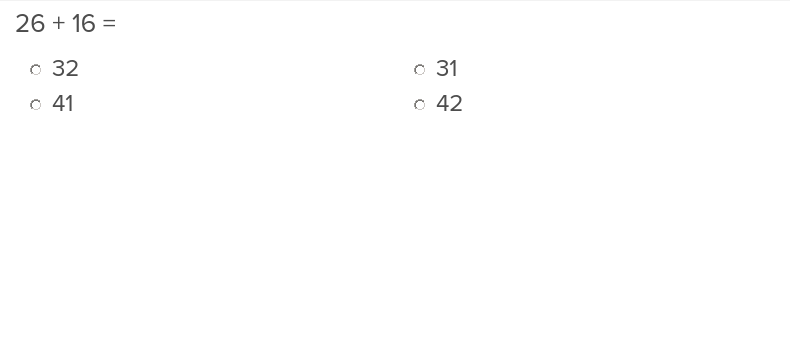Give students an introduction to regrouping that is easy to understand with this two digit addition exercise.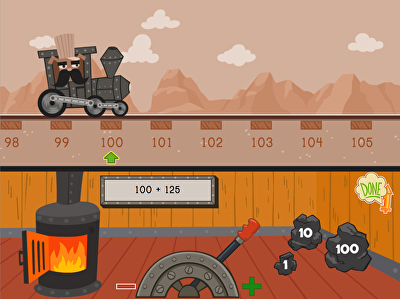This train has got lots of stops to make on the Pacific Railway, and the conductor needs help to keep things chugging along. Kids practice addition on a number line with this fun-filled train game. Players must shovel coal that represent 1, 10, or 100 increments in order to add three-digit numbers. Kids can use addition and subtraction to land on the sum in as few moves as possible.

## Three-Digit Addition on a Number Line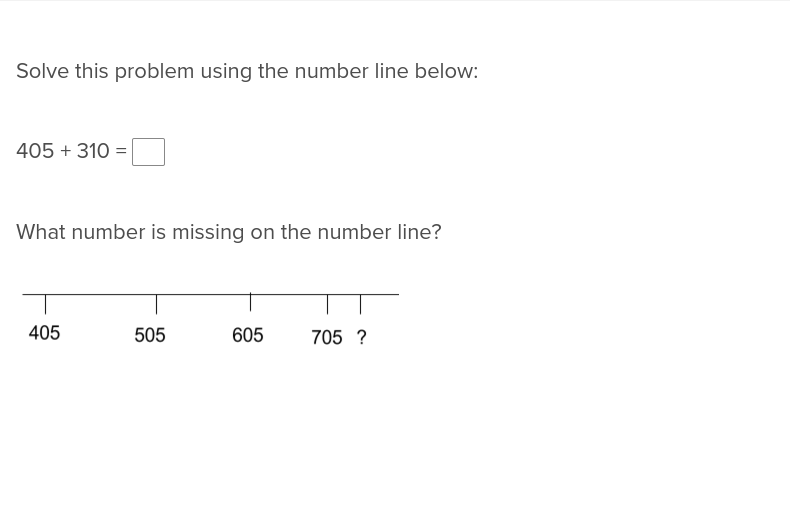Simplify the seemingly complex task of three digit addition with the following number line activity.

## Three-Digit Addition and Regrouping Numbers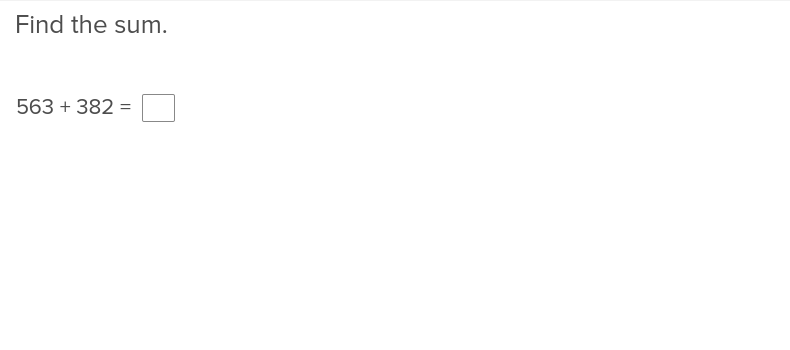Three digit addition just got much simpler thanks to this exercise that illustrates how to regroup numbers.

## Elliott's Museum: Addition and Subtraction Word Problem StoryElliott is opening a museum for all of her favorite things, and needs some help working through the word problems to help her count up the number of items she'll display. Kids practice addition and subtraction with three-digit numbers with these step-by-step instructions and word problems. Using a tape diagram, kids help Elliott sort through her treasures by solving word problems.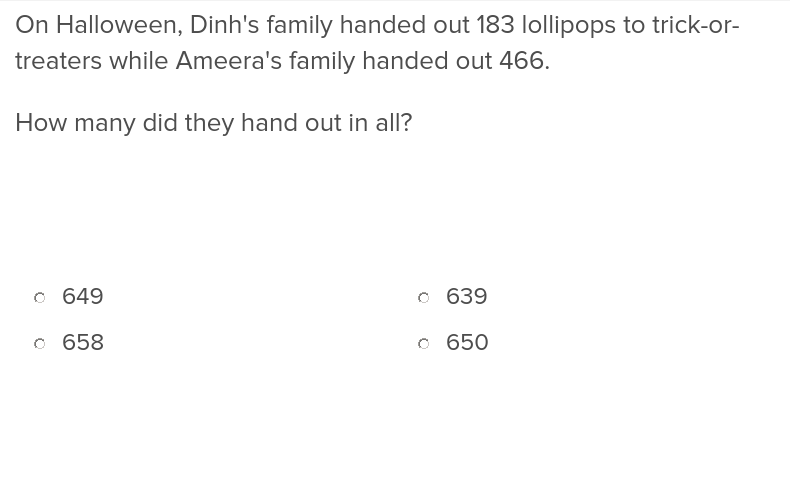Teach students how to extract information from word problems and complete three digit addition to find the solution.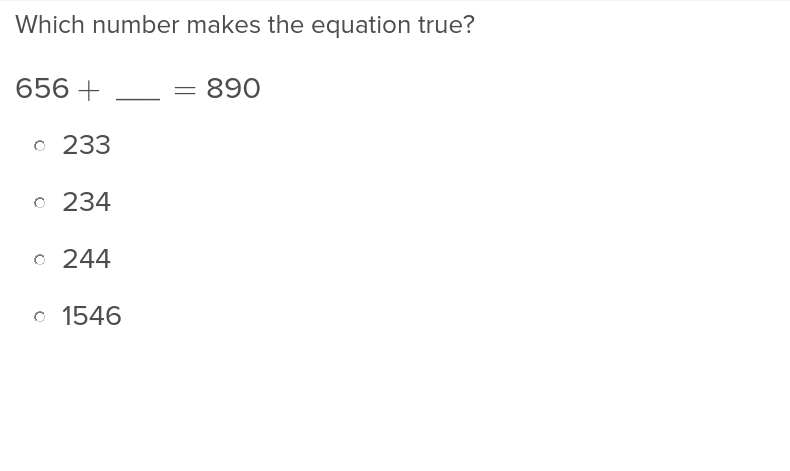Adding up missing factors will be a routine matter for students after they complete this exercise.

## Three-Digit Addition and Two-Step Word Problems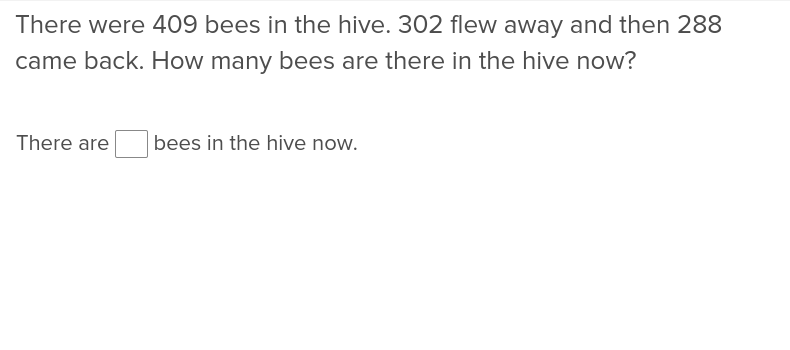Students learning how to perform three digit addition in two step word problems will appreciate the clear instructions in this exercise.